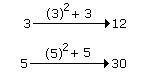# Verbal Reasoning - Analogy

Exercise : Analogy - Analogy 3
Directions to Solve
In each of the following questions find out the alternative which will replace the question mark.

1.
14 : 9 :: 26 : ?
12
13
31
15
Explanation:

14 = (2 x 9 - 4)

26 = (2 x 15 - 4)

? = 15

2.
MO : 13 11 :: HJ : ?
19 17
18 16
8 10
16 18
Explanation:
No answer description is available. Let's discuss.

3.
123 : 132 :: 235 : ?
232
352
253
252
Explanation:

As, 123 → 132

As, 235 → 253

The middle digit of first term becomes power to the next term.

4.
8 : 28 :: 27 : ?
28
8
64
65
Explanation:

First number = 8 and the sum of the digits of the second number is 2 + 8 = 10.

Thus the difference of the first number and the sum of the digits of second number is 10 - 8 = 2.

Similarly, the sum of the digits of third number is 2 + 7 = 9.

Hence the sum of digits of fourth number should be 2 more than 9 i.e. 11

Hence, fourth number is 65.

5.
3 : 12 :: 5 : ?
25
35
30
15